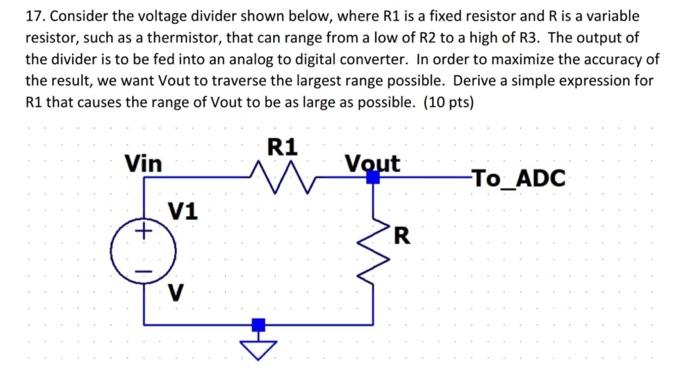Home / Expert Answers / Electrical Engineering / 17-consider-the-voltage-divider-shown-below-where-mathrm-r-1-is-a-fixed-resistor-and-pa777

# (Solved): 17. Consider the voltage divider shown below, where $$\mathrm{R} 1$$ is a fixed resistor and $$\ ...17. Consider the voltage divider shown below, where \( \mathrm{R} 1$$ is a fixed resistor and $$\mathrm{R}$$ is a variable resistor, such as a thermistor, that can range from a low of R2 to a high of R3. The output of the divider is to be fed into an analog to digital converter. In order to maximize the accuracy of the result, we want Vout to traverse the largest range possible. Derive a simple expression for R1 that causes the range of Vout to be as large as possible. (10 pts)

We have an Answer from Expert